+
Solving Linear Equations and Inequalities
0
of 0 possible points

# Properties of Addition and Multiplication

Author: Sophia Tutorial
##### Description:

Identify the commutative property, associative property, or distributive property.

(more)

Sophia’s self-paced online courses are a great way to save time and money as you earn credits eligible for transfer to many different colleges and universities.*

No credit card required

46 Sophia partners guarantee credit transfer.

299 Institutions have accepted or given pre-approval for credit transfer.

* The American Council on Education's College Credit Recommendation Service (ACE Credit®) has evaluated and recommended college credit for 33 of Sophia’s online courses. Many different colleges and universities consider ACE CREDIT recommendations in determining the applicability to their course and degree programs.

Tutorial
what's covered
1. Identity Properties
2. Inverse Properties
3. Commutative Property of Addition and Multiplication
4. Associative Properties of Addition and Multiplication
5. Distributive Property

# 1. Identity Properties

The identity property of addition states that when zero is added to any number, the value does not change. Below is an example of this property using real numbers: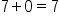In other words, the identity property of addition tells us that adding zero does not change the value of a number. Generally, we can express this as:

formula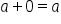A similar property applies to multiplication. What quantity, when multiplied to a number, does not change its value? When any number is multiplied by 1, the value does not change. Let's take a look at a numerical example of the identity property of multiplication: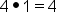The identity property of multiplication states that any number multiplied by 1 does not change in value. Generally, we can express this as:

formula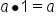# 2. Inverse Properties

The inverse property of addition states that any number and its opposite sum to zero. We can refer to the opposite of a number as its additive inverse. A numerical example is illustrated below:

9 + ( -9 ) = 0

9 and –9 are opposites of each other. This means that their magnitudes are the same (9), but their signs are different; one is a positive number, while the other is a negative number. The sum of a number and its opposite is zero. We can write this generally as:

formula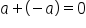The inverse property of multiplication states that a number and its reciprocal multiply to 1. In the same way that a number and its opposite are additive inverses, a number and its reciprocal are multiplicative inverses. The reciprocal of a number can be found by creating a fraction, and flipping the numerator and denominator. Below is a numerical example of the inverse property of multiplication: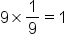9 and 1/9 are multiplicative inverses, or reciprocals, of one another. The inverse property of multiplication dictates that the product of a number and its reciprocal is equal to 1. Here is our general rule:

formula
Inverse Property of Multiplication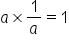# 3. Commutative Property of Addition and Multiplication

Addition and multiplication is commutative. This means that we can add in any order we wish, and we can multiply in any order we wish. It is important to note that we cannot mix addition and multiplication. These are separate properties, but they behave the same with both operations.

term to know
Commutative Property
A property of addition that allows terms to be added in any order; a property of multiplication that allows factors to be multiplied in any order.

Let's take a look at a numerical example of the commutative property of addition: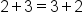The expression 2 + 3 is the same as the expression 3 + 2, because addition is commutative. It does not matter in which order you add, the sum will be 5 in either case.

formula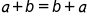The same property applies to multiplication as well. It does not matter in which order you multiply, because multiplication is commutative. A numerical example is illustrated below: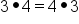The expression 3 x 4 is the same as the expression 4 x 3, because multiplication is commutative. It does not matter in which order you multiply, the product will be 12 in either case.

formula
Commutative Property of Multiplication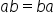# 4. Associative Properties of Addition and Multiplication

The associative property allows us to group terms for addition and multiplication in any way we wish. As with the commutative properties of addition and multiplication, the associative property applies to addition and multiplication separately.

term to know
Associative Property
A property of addition that allows terms to be grouped in any order; a property of multiplication that allows factors to be grouped in any order.

Here are some numerical examples of the associative property:

(3 + 4) + 6 = 3 + (4 + 6)

``` 4 x (2 x 8) = (4 x 2) x 8
```

The associative property allows us to group addends or group factors in different ways. This is particularly helpful in mental math, where we might easily recognize that 4 + 6 is 10. In such cases, regrouping can help us recognize certain sums or products to make mental math easier.

formula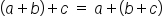formula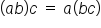# 5. Distributive Property

The distributive property applies multiplication over addition. This property is applied when we have a factor being multiplied by a sum. It got its name from the process of distributing the outside factor into each added of the sum.

term to know
Distributive Property
A property of multiplication that states that a sum multiplied by a factor can be expressed as a sum of the products of each individual addend and that factor.

Let's take a look at a numerical example:

 2 (4 + 3) Distribute 2 into 4 and 3 (2 x 4) + (2 x 3) Multiply inside the parentheses 8 + 6 Add 14 Our solution

formula
Distributive Property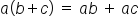hint
The distributive property is especially useful when working with variables. Realistically, an easier approach with numerical examples is to evaluate what is inside the parentheses first, and then multiply the outside factor. (This also follows the Order of Operations). However, as we work with algebraic expressions containing variables, the distributive property is going to be very helpful.
summary
There are many different properties for addition and multiplication. We looked at an identity property, an inverse property, a commutative property, the associative property. Also, for multiplication, there is the distributive property.
Terms to Know
Associative Property

A property of addition that allows terms to be grouped in any order; a property of multiplication that allows factors to be grouped in any order.

Commutative Property

A property of addition that allows terms to be added in any order; a property of multiplication that allows factors to be multiplied in any order.

Distributive Property

A property of multiplication that states that a sum multiplied by a factor can be expressed as a sum of the products of each original addend and that factor.

Formulas to KnowAssociative Property of MultiplicationCommutative Property of MultiplicationDistributive PropertyIdentity Property of Multiplication